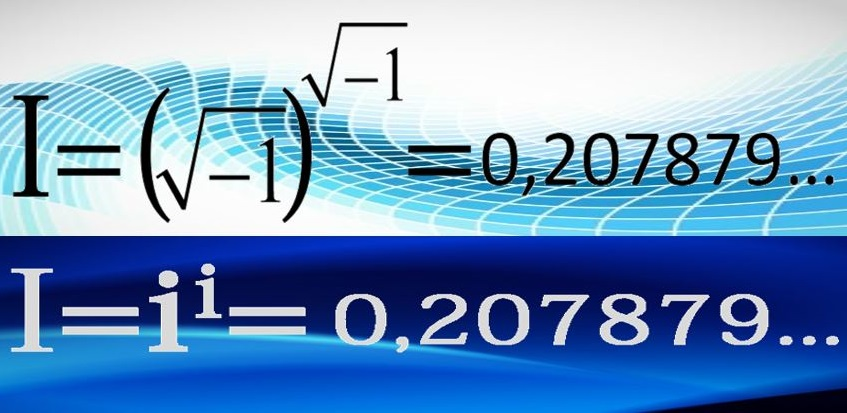# 17 Interesting Facts about Imaginary Numbers

Imaginary numbers are a fascinating mathematical concept that expands our understanding of numbers beyond the realm of real numbers. These numbers are a vital component of the complex number system, a field of mathematics that finds widespread application in various scientific and engineering disciplines.

Imaginary numbers are defined as numbers expressed as a multiple of ‘i,’ where ‘i’ is the imaginary unit representing the square root of -1, written as √(-1). Imaginary numbers are typically represented as ‘bi,’ with ‘b’ being a real number and ‘i’ signifying the imaginary part. This unique definition allows us to work with numbers that have no real counterparts.

When combined with real numbers, imaginary numbers form complex numbers, which are represented in the format ‘a + bi.’ In this representation, ‘a’ is the real part, and ‘b’ is the imaginary part. Complex numbers are invaluable in a wide range of mathematical operations and have numerous practical applications.

One prominent application of imaginary numbers is in the fields of engineering and physics. Complex numbers are used to describe phenomena involving oscillations, alternating current circuits, and wave functions. For instance, in electrical engineering, complex numbers are instrumental in analyzing AC circuits and signal processing, allowing engineers to solve problems related to phase and amplitude.

Imaginary numbers also play a vital role in representing square roots of negative numbers, which are undefined within the real number system. For example, the square root of -9 can be expressed as 3i, signifying that it is a multiple of the imaginary unit. This ability to handle the square roots of negative numbers is particularly significant in fields where mathematical modeling and calculations involve such values.

One common way to visualize imaginary numbers is through the complex plane, where the horizontal axis represents the real part, and the vertical axis signifies the imaginary part. This graphical representation enables a geometric interpretation of complex numbers and their relationships. It provides a powerful tool for solving mathematical problems and analyzing complex phenomena. Imaginary numbers, with their unique properties and applications, enrich the realm of mathematics and contribute to our understanding of the mathematical world.Imaginary Number (Wikimedia)

To know more about imaginary numbers, let’s take a look at these 17 interesting facts about imaginary numbers.

1. Imaginary Unit ‘i’: The imaginary unit ‘i’ is defined as the square root of -1, represented as √(-1).
2. Complex Numbers: Imaginary numbers, when combined with real numbers, form complex numbers in the format ‘a + bi,’ where ‘a’ is the real part and ‘b’ is the imaginary part.
3. Imaginary Roots: Imaginary numbers are used to represent the square roots of negative numbers, which are undefined within the real number system.
4. Complex Plane: Complex numbers, including imaginary numbers, can be graphically depicted on the complex plane, with the real part on the horizontal axis and the imaginary part on the vertical axis.
5. Euler’s Formula: Euler’s formula, e^(iπ) + 1 = 0, is a famous equation that elegantly ties together five of the most important mathematical constants: 0, 1, π, e, and i.
6. Fundamental Theorem of Algebra: The Fundamental Theorem of Algebra states that every non-constant polynomial has at least one complex root, which may be real or imaginary.
7. Imaginary Exponents: Imaginary numbers can be raised to imaginary exponents, leading to complex results with real and imaginary components.
8. Real-Valued Functions: Imaginary numbers are often used in mathematics to simplify real-valued functions and make complex calculations more manageable.
9. Square Roots of -1: Imaginary numbers are the solutions to the equation x^2 = -1, where ‘x’ represents the square root of -1.
10. Notation: Imaginary numbers are typically represented as ‘bi,’ where ‘b’ is a real number and ‘i’ signifies the imaginary part.
11. Quadratic Equations: Imaginary numbers are used to find solutions to quadratic equations with no real roots, known as complex roots.
12. Applications in Electrical Engineering: Complex numbers, which include imaginary components, are crucial in analyzing alternating current (AC) circuits and signal processing.
13. Imaginary and Real Axes: On the complex plane, the imaginary and real axes are perpendicular, forming a coordinate system akin to the Cartesian plane.
14. Conjugate Pairs: Complex numbers come in conjugate pairs, where the real parts are equal, but the imaginary parts have opposite signs.
15. De Moivre’s Theorem: De Moivre’s theorem allows for the calculation of powers and roots of complex numbers, which includes imaginary components.
16. Complex Conjugate: The complex conjugate of a complex number is obtained by changing the sign of its imaginary part while keeping the real part the same.
17. Imaginary Numbers in Quantum Mechanics: Imaginary numbers play a fundamental role in the mathematical underpinnings of quantum mechanics, a branch of physics that describes the behavior of particles at the atomic and subatomic levels.

Imaginary numbers, characterized by the imaginary unit ‘i’ and their role in forming complex numbers, have transcended mathematical abstraction to become indispensable tools in a myriad of scientific, engineering, and mathematical disciplines. They offer solutions to equations with no real roots, provide a unique perspective on the complex plane, and underpin important theorems and formulas. Whether used in solving problems in electrical engineering, understanding wave functions in quantum mechanics, or exploring creative mathematical relationships, imaginary numbers exemplify the boundless nature of mathematical exploration and innovation. Their intriguing presence in the world of numbers has not only expanded our mathematical toolkit but also enriched our comprehension of the underlying structures of reality itself.